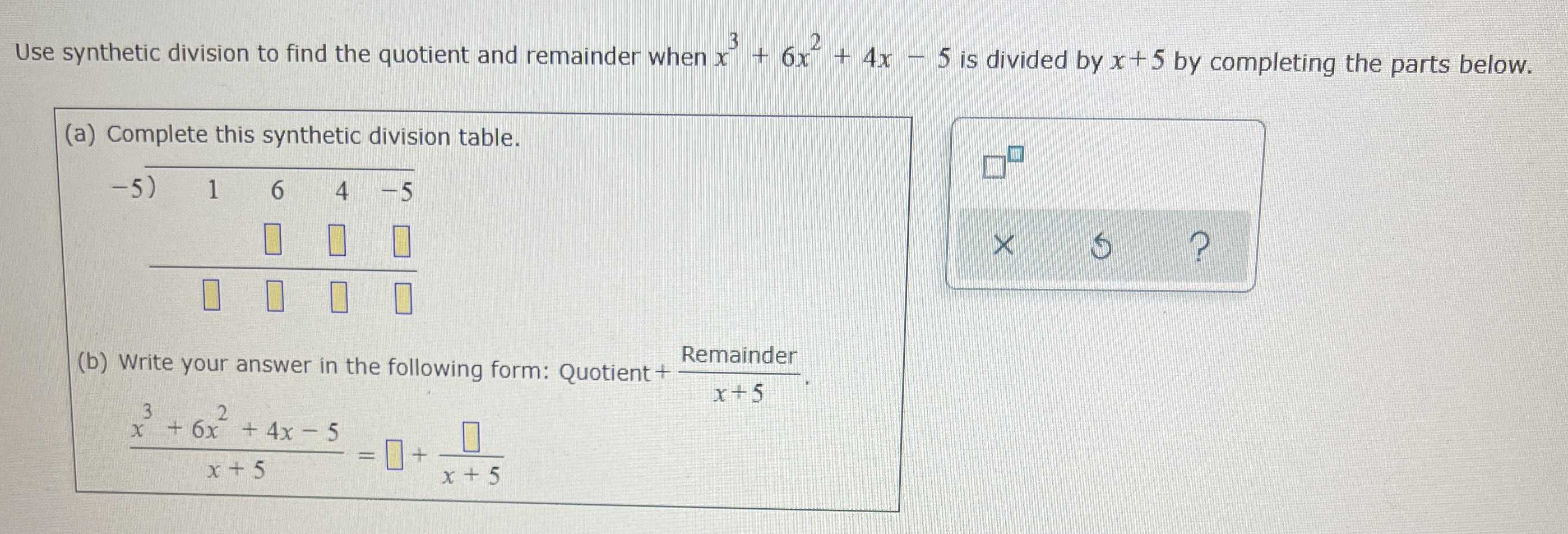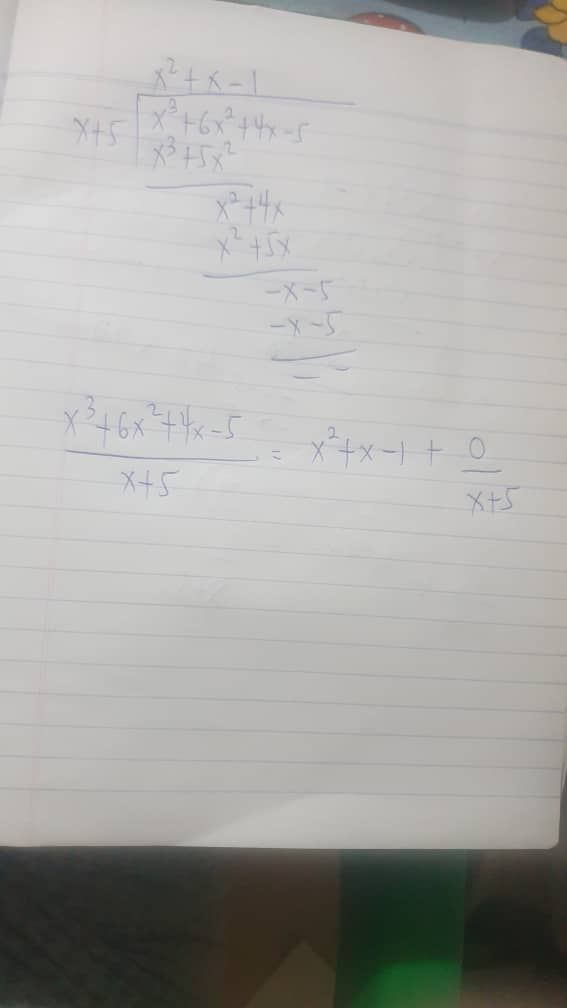### Still have math questions?

Algebra
QuestionUse synthetic division to find the quotient and remainder when $$x ^ { 3 } + 6 x ^ { 2 } + 4 x - 5$$ is divided by $$x + 5$$ by completing the parts below. (a) Complete this synthetic division table. (b) Write your answer in the following form:Quotient + $$\frac{remainder}{x+ 5}$$

$$\frac { x ^ { 3 } + 6 x ^ { 2 } + 4 x - 5 } { x + 5 } = \square + \frac { \square } { x + 5 }$$